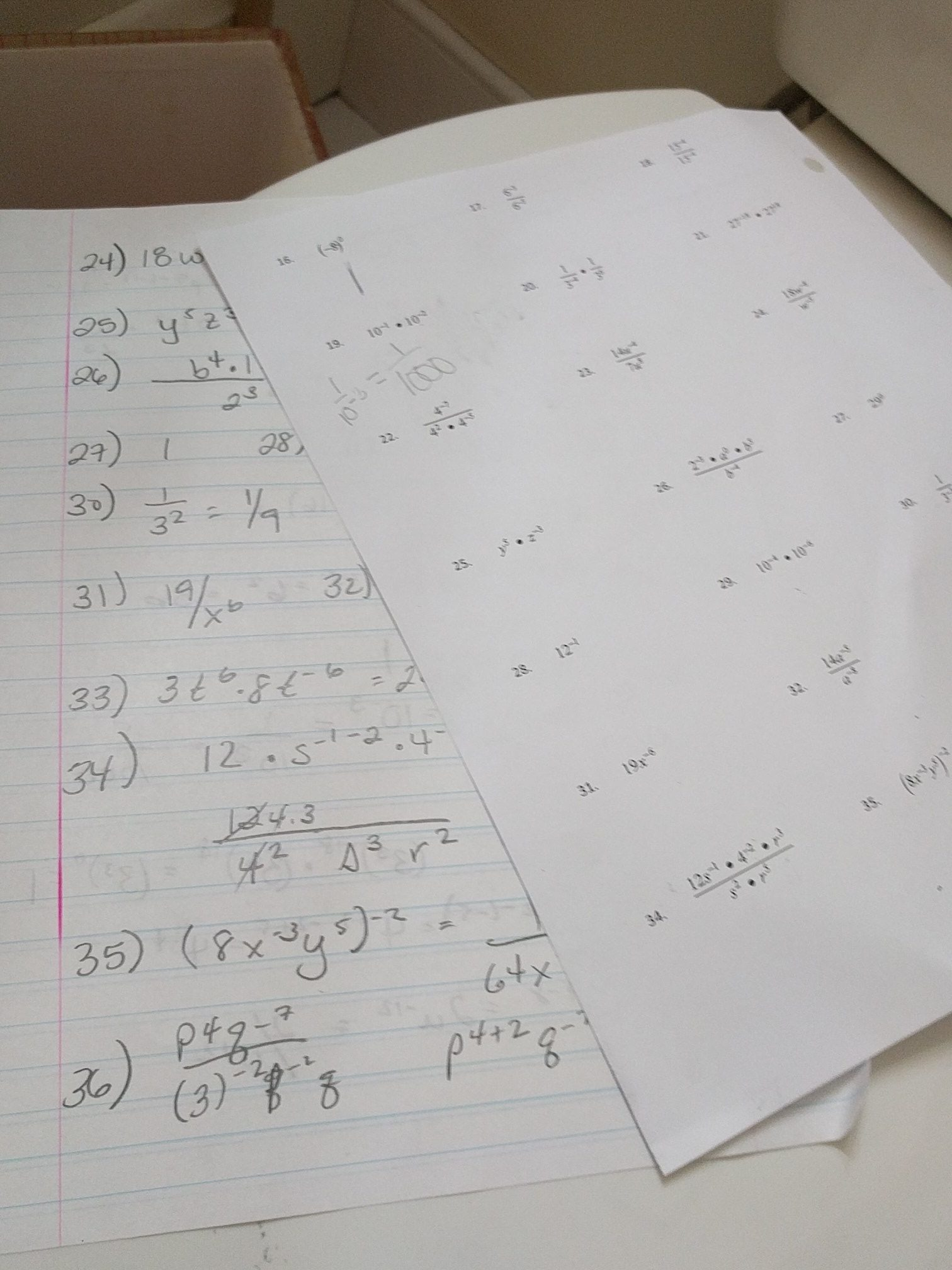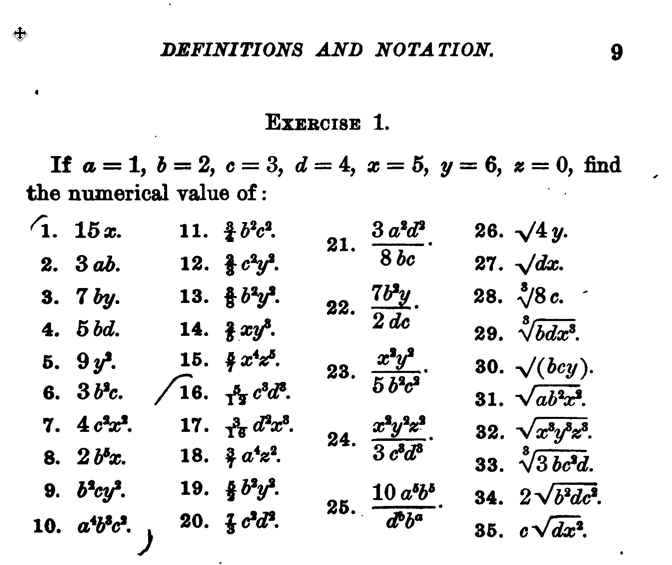Jo Boaler in her book Mathematical Mindsets describes math as “creative and visual.” I have a degree in math, I enjoy doing math problems. What I really enjoy is using my math to creatively solve problems, one of many reasons I have a love affair with spreadsheets. When I create and design a spreadsheet it is likely to not be the exact same spreadsheet someone else would create to tackle the same problem. Sometimes math is taught as the procedural following of steps rather than the creative process of discovery and visualization.

## 1900 Homework

I was at a friends house and saw their child working on a math worksheet for Algebra. THIRTY SIX exponents problems. Take a look at this website with an image of math work from 1898. It is almost exactly the same thing! Over ONE HUNDRED years later and students are doing the same assignments?! Let’s teach like it is 2017 not 1900!

Just for “fun” I cranked out these 36 problems. My hand hurt after finishing them. I would not describe this as an enjoyable activity. Is it any wonder so many students and adults do not like math when they are doing excessive rote math problems rather than engaging in creative and visual activities? If a student does an entire page of problems incorrectly, they have practiced doing it wrong. It is soul sucking to do an entire page of problems and discover that you were not doing it correctly. This is not a confidence builder. If students do understand the problems, an entire page (front and back) quickly becomes busy work. How many problems does a student need to do to demonstrate they get it?

Doing Math in 2017 should be different than 1900.Math homework from 2017Math homework from 1898

I was able to find the entire algebra book from 1898. The problems look the SAME as what my book was using when I taught Algebra.

## Some Considerations

• How are students engaged in the assignment?
• Will the assignment contribute to the student’s passion for the subject?
• Students deserve to be using technology. Technology including Google and YouTube are an undeniable part of everyday life. It does not matter if we are comfortable with technology, we are teaching students who will be using math in the future, not in 1900.
• Are the students using Desmos?
• How will students receive feedback?
• How are students making creative decisions?
• How are students using real data?
Check out Denis Sheeran and his book instant relevance for how he uses things in his life to design math lessons.
• How are students collaborating?
• How are students clearly communicating their ideas? This does not mean show your steps.
• How are students using critical thinking? This worksheet is DOK 1, not critical thinking. All procedural step following.
• What context is presented?
• Which of the 8 mathematical principles are the students demonstrating?
Note: Show your steps is NOT one of the mathematical principles.
• What authentic audience does the student have?
• How long will it take the student to complete the task?
• How does the assignment differentiate for struggling and advanced students?
• How will this assignment conflict with work required for other classes?
• How will this assignment impact family time?
• Will this assignment possibly contribute to family stress and fighting?

## 3 Math Problems

In her book Mathematical Mindsets, Jo Boaler says that doing repetitive problems is not helpful. If doing 30 problems worked everyone would be awesome at math. After a couple of problems you’re not critically thinking about the problems. Compare and contrast solutions to 3 problems. Model 3 problems. Analyze over memorize. Do less but go deeper. Students need to think about what they are doing and understand it. Showing steps does not demonstrate an understanding of the math, only an ability to follow directions.

Offer challenges, not necessarily more math problems.

Choice is very important. Instead of having all students doing the same page of math problems, allow them to choose 3 problems. Students should model, explain, and demonstrate thinking (again, going beyond showing procedural steps … which by the way can be copied down from wolframalpha.com or their neighbor.) Provide students high quality feedback conversations (Google Slides is great for this!) to help students to understand what they are doing rather than just marking the correct answer. When students realize they are not just doing math to get their homework done, but rather have an opportunity to learn and expand their thinking, students switch from choosing the easy problems to the most difficult problems.

Make sure you check out openmiddle.com for DOK2 and DOK3 math problems. Here is a math puzzle I adapted from the website. Notice the menu at the top to allow you to create new puzzles. Here is a link to an order of operations activity in Google Slides. Students think about the math rather than simply doing the math. This count the dots activity was also adapted from Open Middle. The focus is not on the answer, but rather the STRATEGY the student utilized.
Also check out robertkaplinsky.com to help with increasing critical thinking for math!

## Jobs of the Future

Watching my friends kids do their homework looked like they were being prepared for boring clerical jobs.

Jobs of the future will not require computation. They will require creativity and critical thinking. Robots will follow procedural steps. Heck, we won’t even be driving our own cars.  The skill then becomes how do you fix something when it breaks? Skills from 1900 will not be as valuable in 2046. How are we preparing students for their future?## Subscribe to the Alice Keeler Newsletter